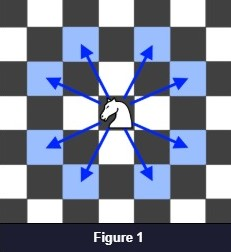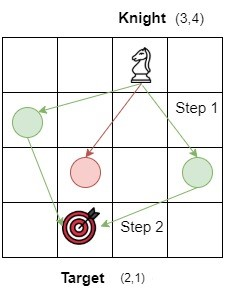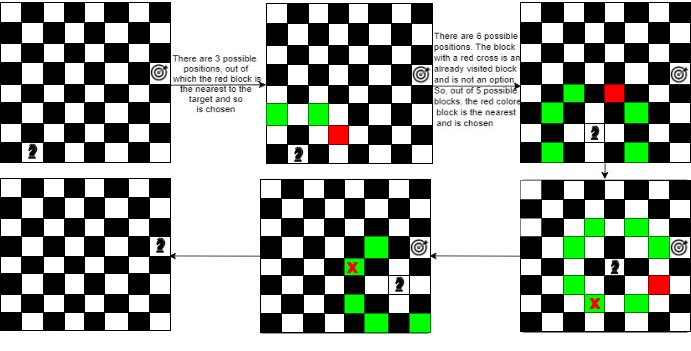Problem title
Difficulty
Avg time to solve

Sorted Matrix
Moderate
25 mins
Moderate
35 mins
Odd even level
Easy
20 mins
Left And Right Rotation Of A String
Easy
15 mins
Sorted Linked List to Balanced BST
Moderate
25 mins
Max element in the path
Easy
15 mins
Count Triplets
Easy
15 mins
Given a string, find the next smallest palindrome
Easy
12 mins
Minimum Characters For Palindrome
Hard
20 mins
Minimum steps to reach target by a Knight
Moderate
25 mins14

# Minimum steps to reach target by a Knight

Difficulty: MEDIUM
Avg. time to solve
25 min
Success Rate
60%

Problem Statement

#### Your task is to find out the minimum steps a Knight will take to reach the target position.##### Example:
``````knightPosition: {3,4}
targetPosition: {2,1}
````````````The knight can move from position (3,4) to positions (1,3), (2,2) and (4,2). Position (4,2) is selected and the ‘stepCount’ becomes 1. From position (4,2), the knight can directly jump to the position (2,1) which is the target point and ‘stepCount’ becomes 2 which is the final answer.
``````

#### Note:

``````1. The coordinates are 1 indexed. So, the bottom left square is (1,1) and the top right square is (N, N).

2. The knight can make 8 possible moves as given in figure 1.

3. A Knight moves 2 squares in one direction and 1 square in the perpendicular direction (or vice-versa).
``````

#### Input format:

``````The first line of input contains an integer ‘T’ denoting the number of test cases.
The next ‘3*T’ lines represent the ‘T’ test cases.

The first line of each test case contains a pair of integers denoting the initial position of the knight.

The second line of each input contains a pair of integers denoting the target position.

The third line of each test case contains an integer ‘N’ denoting the rows/columns of the chessboard.
``````

#### Output format:

``````For each test case, print an integer representing the minimum steps a Knight will take to reach the target position.
``````
##### Note :
``````You do not need to print anything; it has already been taken care of. Just implement the given function.
``````

#### Constraints

``````1 <= T <= 10
1 <= N <= 1000
1 <= KNIGHTPOSITION(X, Y), TARGETPOSITION(X, Y) <= N

Time limit: 1 second
``````

#### Sample input 1

``````2
8
2 1
8 5
6
4 5
1 1
``````

#### Sample output 1

``````4
3
``````

#### Explanation of sample input 1:

``````Test case 1:
````````````The knight is initially at position [2,1]. It has 3 possible blocks to move to, [4,2], [3,3], and [1,3]. The knight chooses [4,2]. Now, there are 6 more possible blocks to move to. The knight chooses [5,4]. Further, the knight chooses position [7,3]. Finally, the knight moves to the target position which is [8,5] which calculates to 4 steps.

Test case 2:
The knight moves from position [4,5] to [5,3] to [3,2] and finally to the target position [1,1] which gives us the minimum steps by the knight, that is, 3.
(4, 5) -> (5, 3) -> (3, 2) -> (1, 1).
``````

#### Sample input 2:

``````2
6
2 4
6 4
6
1 1
4 5
``````

#### Sample output 2:

``````2
3
``````Console